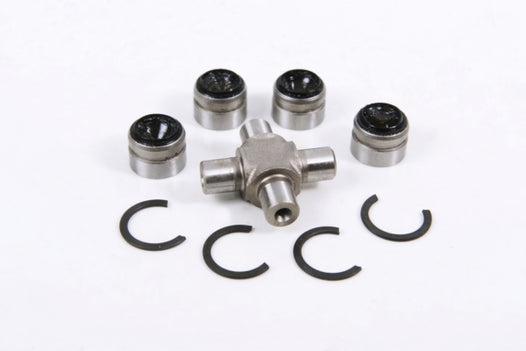Quick Directory
• Products

#### Winter Accessories# EPI Universal Joint (Tip length: )

EPI

05-294408

High quality Grease zerks on most Product Numbers294400Tip length: Total length: Zerk: NoSnap ring included: YesPosition: Varies by modelModel number: WE100200 294401Tip length: Total length: Zerk: YesSnap ring included: YesPosition: Varies by modelModel number: WE100210 294402Tip length: Total length: Zerk: YesSnap ring included: YesPosition: Varies by modelModel number: WE100220 294403Tip...
Regular price \$26.99
• High quality
• Grease zerks on most
##### Product Numbers

294400
Tip length:
Total length:
Zerk: No
Snap ring included: Yes
Position: Varies by model
Model number: WE100200

294401
Tip length:
Total length:
Zerk: Yes
Snap ring included: Yes
Position: Varies by model
Model number: WE100210

294402
Tip length:
Total length:
Zerk: Yes
Snap ring included: Yes
Position: Varies by model
Model number: WE100220

294403
Tip length:
Total length:
Zerk: Yes
Snap ring included: Yes
Position: Varies by model
Model number: WE100223

294405
Tip length:
Total length:
Zerk: Yes
Snap ring included: Yes
Position: Varies by model
Model number: WE100270

294406
Tip length:
Total length:
Zerk: Yes
Snap ring included: Yes
Position: Varies by model
Model number: WE100280

294407
Tip length:
Total length:
Zerk: Yes
Snap ring included: Yes
Position: Varies by model
Model number: WE100285

294408
Tip length:
Total length:
Zerk: Yes
Snap ring included: Yes
Position: Varies by model
Model number: WE100290

294409
Tip length:
Total length:
Zerk: Yes
Snap ring included: Yes
Position: Varies by model
Model number: WE100338

294410
Tip length:
Total length:
Zerk: Yes
Snap ring included: Yes
Position: Varies by model
Model number: WE100395

294411
Tip length:
Total length:
Zerk: Yes
Snap ring included: Yes
Position: Varies by model
Model number: WE100507

294412
Tip length:
Total length:
Zerk: Yes
Snap ring included: Yes
Position: Varies by model
Model number: WE100510

294414
Tip length:
Total length:
Zerk: Yes
Snap ring included: Yes
Position: Varies by model
Model number: WE100600

294415
Tip length:
Total length:
Zerk: Yes
Snap ring included: Yes
Position: Varies by model
Model number: WE100811

294416
Tip length:
Total length:
Zerk: Yes
Snap ring included: Yes
Position: Varies by model
Model number: WE100995

294702
Tip length:
Total length:
Zerk: Yes
Snap ring included: Yes
Position: Varies by model
Model number: WE100230

294707
Tip length:
Total length:
Zerk: Yes
Snap ring included: Yes
Position: Varies by model
Model number: WE100603

294708
Tip length:
Total length:
Zerk: Yes
Snap ring included: Yes
Position: Varies by model
Model number: WE100996

294709
Tip length:
Total length:
Zerk: Yes
Snap ring included: Yes
Position: Varies by model
Model number: WE100997

294695
Tip length:
Total length:
Zerk: Yes
Snap ring included: Yes
Position: Varies by model
Model number: WE100227

295417
Tip length:
Total length:
Zerk: Yes
Snap ring included: Yes
Position: Varies by model
Model number: WE100999